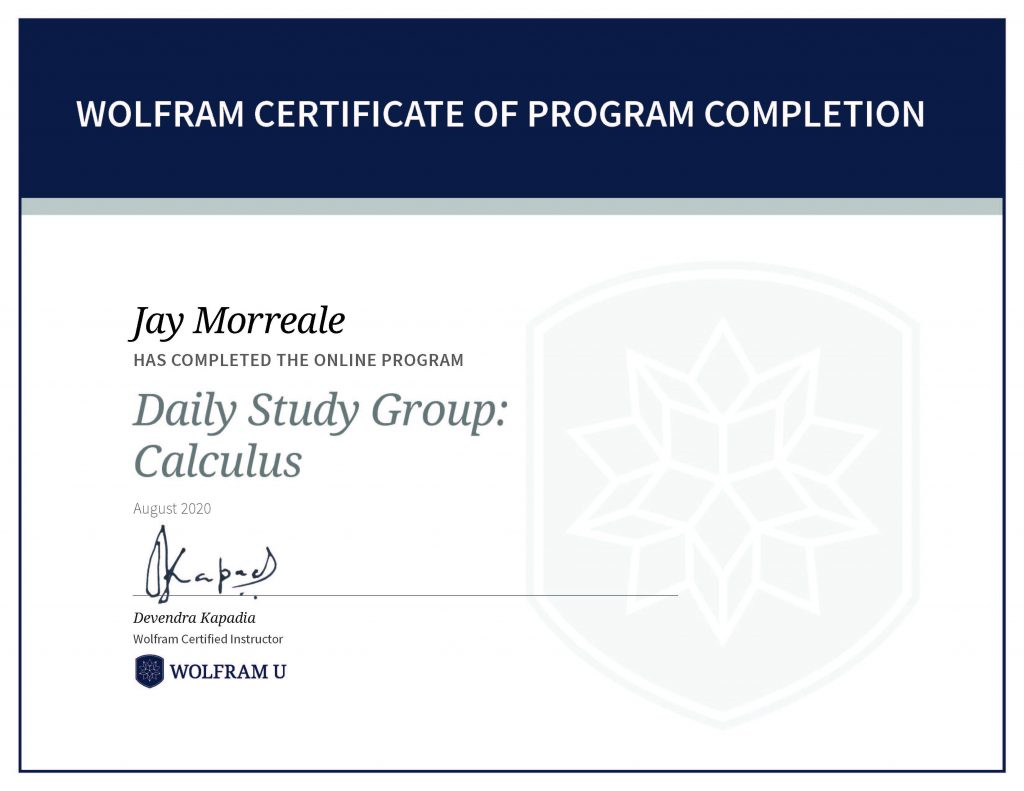# Wolfram U Daily Study Group: Calculus

The three week Calculus Study Group (4) uses the Introduction to Calculus self study course as part of the daily lectures and covers functions, limits, differential calculus, and integral calculus. This course is a good way to prepare for AP Calculus and is a great refresher on the topic.

The functions 3x, the derivative of 3x, ex, the derivative of ex, and 2x, the derivative of 2x are shown in the plot on the left in the figure below to show the awesome properties of ex, for example. The derivative of ex is ex so the slope anywhere on the line is just the value of the function at that point.

The tangent line and normal line are shown in the plot on the right for ex=3. This point was chosen as an example. The functions used to create the tangent and normal lines are as follows.

• f(x)=ex
• Tangent(x)=f′(x)(x−x0)+f(x0) at x0=3
• Normal(x)=(−1/f′(x))(x−x0)+f(x0) at x0=3

The area under ex to x0=3 can be found by integrating the curve from −∞ to 3 and is e3. Thus, the area under the curve is also the value of the function at that point.

To receive a program completion certificate you must pass three quizzes.Wolfram Certificate of Program Completion, Jay Morreale has completed the online program Daily Study Group: Calculus.